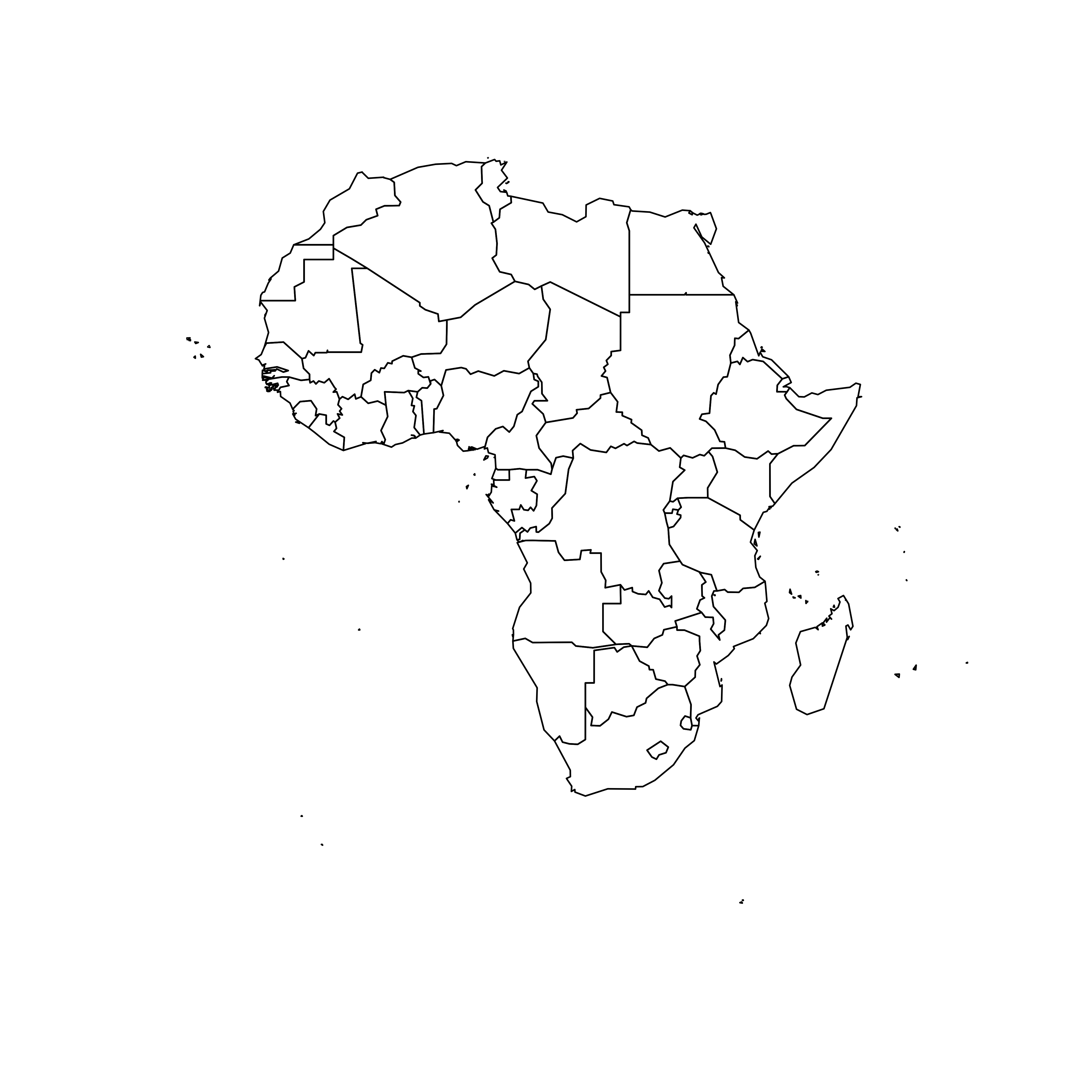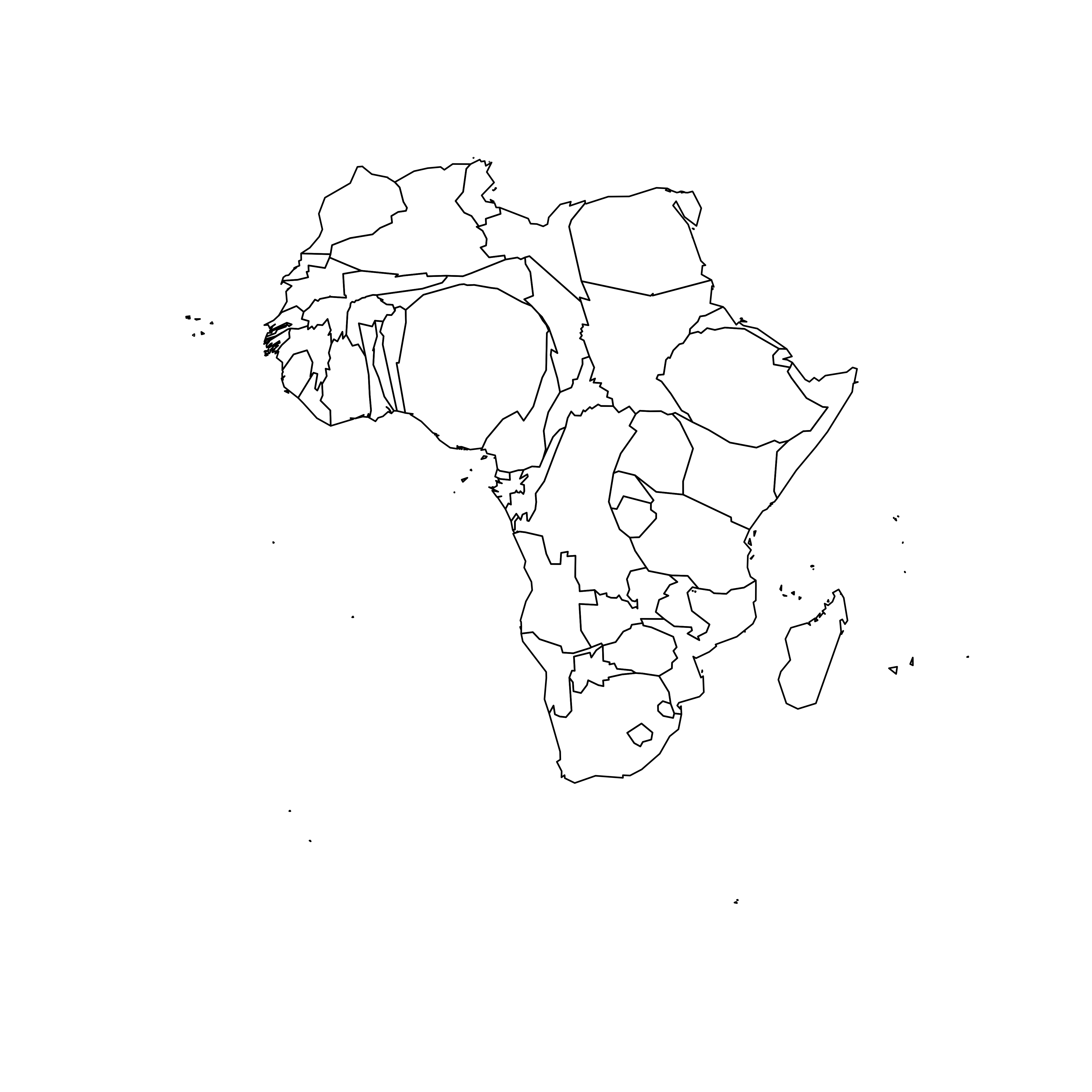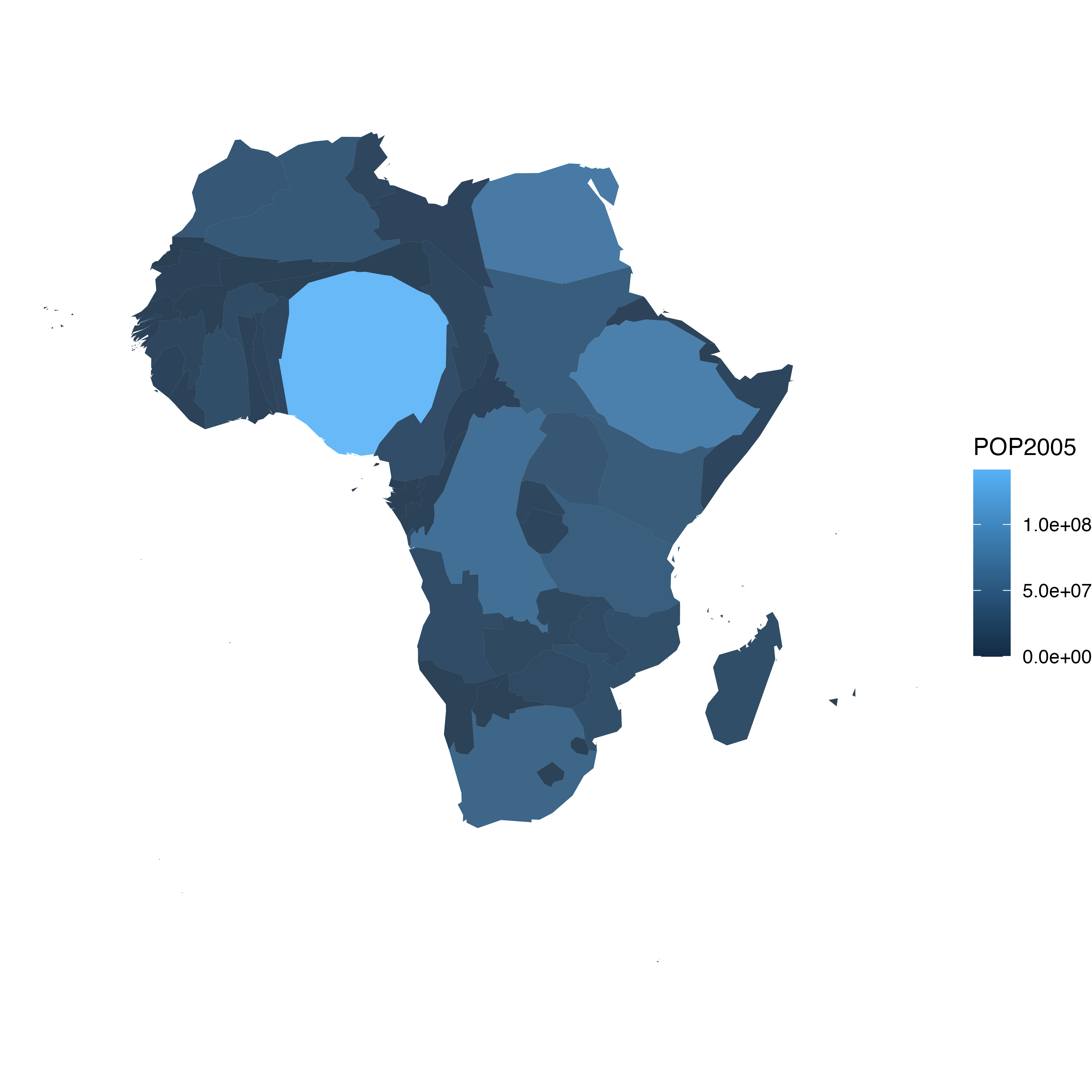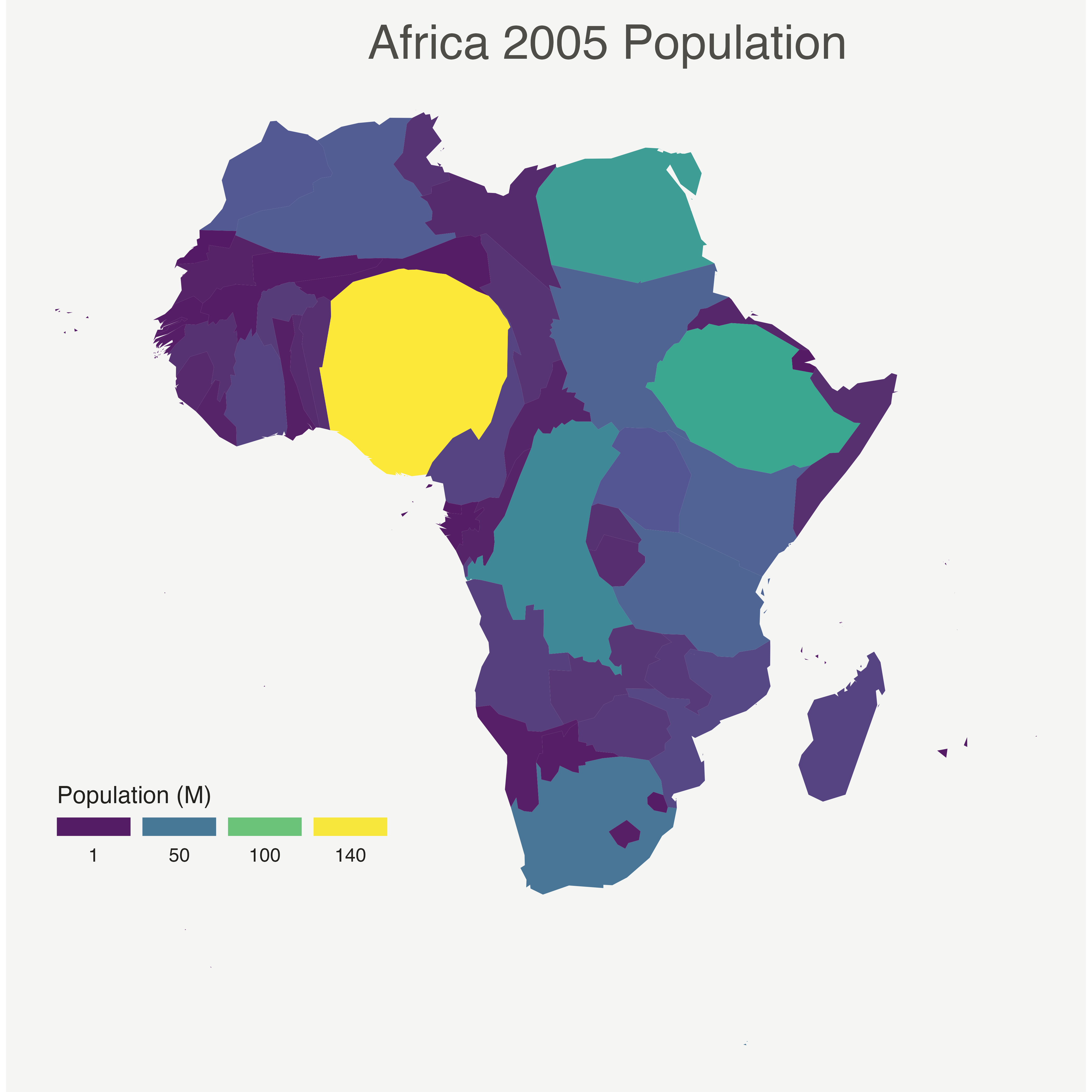# Basic Cartogram with R

This post descriibes how to build a very simple cartogram with `R` and the `cartogram` package. It is a step a step approach leading to a choropleth map with distorted region size.

# Very basic map

A cartogram is a map in which the geometry of regions is distorted in order to convey the information of an alternate variable. In this post, we are going to draw a map of Africa where the size of each country is distorted proportionally to its population.

First of all, you need to understand what a geospatial object is, and how to plot it with `R`. See the background map section of the gallery!

Let’s use the `maptools` library which provides a geospatial object with the Africa’s boundaries. Note that you can get a similar object from a shapefile, or from a geojson file!

We can plot the boundaries using the `plot()` function:``````# Get the shape file of Africa
library(maptools)
data(wrld_simpl)
afr=wrld_simpl[wrld_simpl\$REGION==2,]

# We can visualize the region's boundaries with the plot function
plot(afr)``````

# Distort country size with `cartogram`

The geospatial object has a `data slot`: an attached data frame that provides several information for each region. It notably gives the population of each country in 2005.

We can thus use the `cartogram` library to distort the size of each country, proportionally to this column. The new geospatial object we get can be draw with the same `plot` function!

See how Nigeria is now bigger?``````# We work with the cartogram library
library(cartogram)

# construct a cartogram using the population in 2005
afr_cartogram <- cartogram(afr, "POP2005", itermax=5)

# This is a new geospatial object, we can visualise it!
plot(afr_cartogram)``````

# Cartogram and choropleth

Cartogram are very often used in combination with choropleth map. Since we have a spatial object and a numeric value associated with each region, it is possible to color each region according to its value.

Let’s use ggplot2 to add some color, title, legend, background and so on. We now have a nice cartogram chloropleth map of Africa!``````# It is a new geospatial object: we can use all the usual techniques on it! Let's start with a basic ggplot2 chloropleth map:
library(tidyverse)
library(broom)
spdf_fortified <- tidy(afr_cartogram)
spdf_fortified = spdf_fortified %>% left_join(. , afr_cartogram@data, by=c("id"="ISO3"))
ggplot() +
geom_polygon(data = spdf_fortified, aes(fill = POP2005, x = long, y = lat, group = group) , size=0, alpha=0.9) +
coord_map() +
theme_void()``````

# Make it pretty

Same thing with a tiny bit of customization. See more explanation in the choropleth an ggplot2 sections.``````# As seen before, we can do better with a bit of customization
library(viridis)
ggplot() +
geom_polygon(data = spdf_fortified, aes(fill = POP2005/1000000, x = long, y = lat, group = group) , size=0, alpha=0.9) +
theme_void() +
scale_fill_viridis(name="Population (M)", breaks=c(1,50,100, 140), guide = guide_legend( keyheight = unit(3, units = "mm"), keywidth=unit(12, units = "mm"), label.position = "bottom", title.position = 'top', nrow=1)) +
labs( title = "Africa 2005 Population" ) +
ylim(-35,35) +
theme(
text = element_text(color = "#22211d"),
plot.background = element_rect(fill = "#f5f5f4", color = NA),
panel.background = element_rect(fill = "#f5f5f4", color = NA),
legend.background = element_rect(fill = "#f5f5f4", color = NA),
plot.title = element_text(size= 22, hjust=0.5, color = "#4e4d47", margin = margin(b = -0.1, t = 0.4, l = 2, unit = "cm")),
legend.position = c(0.2, 0.26)
) +
coord_map()``````

Related chart types

## Contact

This document is a work by Yan Holtz. Any feedback is highly encouraged. You can fill an issue on Github, drop me a message on Twitter, or send an email pasting yan.holtz.data with gmail.com.